Ch 1. Particle General Motion Multimedia Engineering Dynamics Position,Vel & Accel. Accel. varyw/ Time Accel. Constant Rect. Coordinates Norm/Tang. Coordinates Polar Coordinates RelativeMotion
 Chapter - Particle - 1. General Motion 2. Force & Accel. 3. Energy 4. Momentum - Rigid Body - 5. General Motion 6. Force & Accel. 7. Energy 8. Momentum 9. 3-D Motion 10. Vibrations Appendix Basic Math Units Basic Equations Sections Search eBooks Dynamics Fluids Math Mechanics Statics Thermodynamics Author(s): Kurt Gramoll ©Kurt GramollDYNAMICS - THEORY

Velocity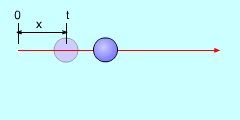Average Velocity

A particle moving in a straight line is generally called rectilinear motion. The displacement, Δx, measures the change in position from a fixed origin O along the straight line. In this discussion, distance is represented by 'x', but could also be 'y', 'z' or even 's' (generic symbol for distance).

The average velocity of the particle is defined as the quotient of the displacement Δx and the time interval Δt over which the displacement takes place.

Average Velocity = Δx/Δt

The instantaneous velocity at time t is defined as the limit as Δt approaches 0 of the average velocity.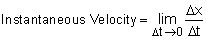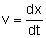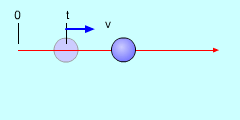Average Acceleration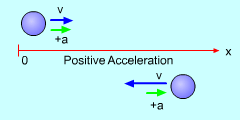Positive Acceleration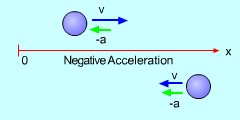Negative Acceleration

Acceleration

The average acceleration is defined as the quotient of the change in velocity Δv and the time interval Δt over which the change in velocity takes place.

Average Acceleration = Δv/Δt

The instantaneous acceleration at time t is defined as the limit as Δt approaches 0 of the average acceleration.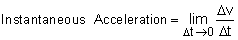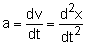For a positive acceleration, either

1. The velocity increases in the positive direction.
2. The velocity decreases in the negative direction.

For a negative acceleration, either

1. The velocity decreases in the positive direction.
2. The velocity increases in the negative direction.

Graphic Analysis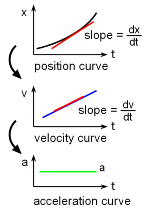If the displacement, velocity, and acceleration are graphed vs. time then

• the slope of Δx vs. Δt at time t equals the velocity at time t, and
• the slope of Δv vs. Δt at time t equals the acceleration at time t.

Practice Homework and Test problems now available in the 'Eng Dynamics' mobile app
Includes over 400 problems with complete detailed solutions.
Available now at the Google Play Store and Apple App Store.